# integral calculus

Also found in: Dictionary, Thesaurus, Medical, Wikipedia.
Related to integral calculus: differential calculus

## calculus

calculus, branch of mathematics that studies continuously changing quantities. The calculus is characterized by the use of infinite processes, involving passage to a limit—the notion of tending toward, or approaching, an ultimate value. The English physicist Isaac Newton and the German mathematician G. W. Leibniz, working independently, developed the calculus during the 17th cent. The calculus and its basic tools of differentiation and integration serve as the foundation for the larger branch of mathematics known as analysis. The methods of calculus are essential to modern physics and to most other branches of modern science and engineering.

### The Differential Calculus

The differential calculus arises from the study of the limit of a quotient, Δyx, as the denominator Δx approaches zero, where x and y are variables. y may be expressed as some function of x, or f(x), and Δy and Δx represent corresponding increments, or changes, in y and x. The limit of Δyx is called the derivative of y with respect to x and is indicated by dy/dx or Dxy:

The symbols dy and dx are called differentials (they are single symbols, not products), and the process of finding the derivative of y=f(x) is called differentiation. The derivative dy/dx=df(x)/dx is also denoted by y′, or f′(x). The derivative f′(x) is itself a function of x and may be differentiated, the result being termed the second derivative of y with respect to x and denoted by y″, f″(x), or d2y/dx2. This process can be continued to yield a third derivative, a fourth derivative, and so on. In practice formulas have been developed for finding the derivatives of all commonly encountered functions. For example, if y=xn, then y′=nxn − 1, and if y=sin x, then y′=cos x (see trigonometry). In general, the derivative of y with respect to x expresses the rate of change in y for a change in x. In physical applications the independent variable (here x) is frequently time; e.g., if s=f(t) expresses the relationship between distance traveled, s, and time elapsed, t, then s′=f′(t) represents the rate of change of distance with time, i.e., the speed, or velocity.

Everyday calculations of velocity usually divide the distance traveled by the total time elapsed, yielding the average velocity. The derivative f′(t)=ds/dt, however, gives the velocity for any particular value of t, i.e., the instantaneous velocity. Geometrically, the derivative is interpreted as the slope of the line tangent to a curve at a point. If y=f(x) is a real-valued function of a real variable, the ratio Δyx=(y2 − y1)/(x2 − x1) represents the slope of a straight line through the two points P (x1,y1) and Q (x2,y2) on the graph of the function. If P is taken closer to Q, then x1 will approach x2 and Δx will approach zero. In the limit where Δx approaches zero, the ratio becomes the derivative dy/dx=f′(x) and represents the slope of a line that touches the curve at the single point Q, i.e., the tangent line. This property of the derivative yields many applications for the calculus, e.g., in the design of optical mirrors and lenses and the determination of projectile paths.

### The Integral Calculus

The second important kind of limit encountered in the calculus is the limit of a sum of elements when the number of such elements increases without bound while the size of the elements diminishes. For example, consider the problem of determining the area under a given curve y=f(x) between two values of x, say a and b. Let the interval between a and b be divided into n subintervals, from a=x0 through x1, x2, x3, … xi − 1, xi, … , up to xn=b. The width of a given subinterval is equal to the difference between the adjacent values of x, or Δxi=xi − xi − 1, where i designates the typical, or ith, subinterval. On each Δxi a rectangle can be formed of width Δxi, height yi=f(xi) (the value of the function corresponding to the value of x on the right-hand side of the subinterval), and area ΔAi=f(xixi. In some cases, the rectangle may extend above the curve, while in other cases it may fail to include some of the area under the curve; however, if the areas of all these rectangles are added together, the sum will be an approximation of the area under the curve.

This approximation can be improved by increasing n, the number of subintervals, thus decreasing the widths of the Δx's and the amounts by which the ΔA's exceed or fall short of the actual area under the curve. In the limit where n approaches infinity (and the largest Δx approaches zero), the sum is equal to the area under the curve:

The last expression on the right is called the integral of f(x), and f(x) itself is called the integrand. This method of finding the limit of a sum can be used to determine the lengths of curves, the areas bounded by curves, and the volumes of solids bounded by curved surfaces, and to solve other similar problems.

An entirely different consideration of the problem of finding the area under a curve leads to a means of evaluating the integral. It can be shown that if F(x) is a function whose derivative is f(x), then the area under the graph of y=f(x) between a and b is equal to F(b) − F(a). This connection between the integral and the derivative is known as the Fundamental Theorem of the Calculus. Stated in symbols:

The function F(x), which is equal to the integral of f(x), is sometimes called an antiderivative of f(x), while the process of finding F(x) from f(x) is called integration or antidifferentiation. The branch of calculus concerned with both the integral as the limit of a sum and the integral as the antiderivative of a function is known as the integral calculus. The type of integral just discussed, in which the limits of integration, a and b, are specified, is called a definite integral. If no limits are specified, the expression is an indefinite integral. In such a case, the function F(x) resulting from integration is determined only to within the addition of an arbitrary constant C, since in computing the derivative any constant terms having derivatives equal to zero are lost; the expression for the indefinite integral of f(x) is

The value of the constant C must be determined from various boundary conditions surrounding the particular problem in which the integral occurs. The calculus has been developed to treat not only functions of a single variable, e.g., x or t, but also functions of several variables. For example, if z=f(x,y) is a function of two independent variables, x and y, then two different derivatives can be determined, one with respect to each of the independent variables. These are denoted by ∂z/∂x and ∂z/∂y or by Dxz and Dyz. Three different second derivatives are possible, ∂2z/∂x2, ∂2z/∂y2, and ∂2z/∂xy=∂2z/∂yx. Such derivatives are called partial derivatives. In any partial differentiation all independent variables other than the one being considered are treated as constants.

### Bibliography

See R. Courant and F. John, Introduction to Calculus and Analysis, Vol. I (1965); M. Kline, Calculus: An Intuitive and Physical Approach (2 vol., 1967); G. B. Thomas and R. L. Finney, Calculus and Analytic Geometry (7th ed. 2 vol., 1988).

The following article is from The Great Soviet Encyclopedia (1979). It might be outdated or ideologically biased.

## Integral Calculus

a branch of mathematics that studies the properties of and methods of computing integrals and their applications. The integral calculus is closely connected with the differential calculus and together with the latter constitutes one of the fundamental parts of mathematical analysis (or the analysis of infinitesimals). Central to the integral calculus are the concepts of the definite integral and indefinite integral of a function of a single real variable.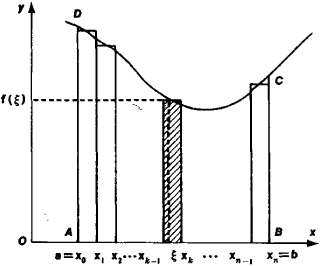Figure 1

Definite integral. Suppose we are required to compute the area S of a “curvilinear trapezoid”—figure ABCD (see Figure 1)— bounded by the continuous curve y = f (x), by the interval AB on the axis of the abscissas, and by the two ordinates AD and BC. In order to calculate the area S, we divide the base AB (the interval [a,b]) into n subintervals (not necessarily equal) by means of points a = x0 < x1 < … < xn. 1 < xn = b, and designate their lengths by Δx1, Δx2, …, Δxn. On each of these subintervals we construct rectangles with altitudes f1), f2),…, fn), where ξk is a point in the subinterval [xk1,xk] (in Figure 1 the rectangle on the k th subinterval is shaded; fk) is its altitude). The sum Sn of the areas of the rectangles is regarded as an approximation to the area 5 of the curvilinear trapezoid:

s≈ sn = f1)Δx1 + f2)Δx2 + … + fn)Δxn

Using the summation symbol Σ (the Greek letter “sigma”) we write, for brevity,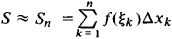The smaller the lengths Δxk of the subintervals, the more exact is the above expression for the area of the curvilinear trapezoid. In order to find the exact value of the area S, it is necessary to find the limit of the sums Sn as the number of points of subdivision increases without bound and the largest of the lengths Δxk tends to zero.

Setting aside the geometric content of our considerations, we arrive at the concept of the definite integral of a function f(x), continuous on the interval [a, b], as the limit, in the sense just described, of the integral sums Sn. This integral is denoted by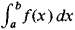The symbol J (an elongated S—the first letter of the word summa) is called the integral sign,f(x) is the integrand, and the numbers a and b are the lower and upper limits of the definite integral. If a = b, then by definition,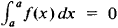Again, by definition,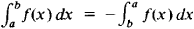The following are properties of the definite integral: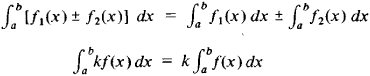where k is a constant. It is also evident that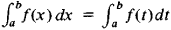(The numerical value of the definite integral does not depend on the choice of designation for the variable of integration.)

Problems of finding areas bounded by curves (“quadrature” problems), lengths of arcs of curves (“rectification of curves”), surface areas of solids, and volumes of solids (“cubature” calculations) are all reduced to the calculation of definite integrals as are problems of determining the coordinates of centers of gravity, moments of inertia, the trajectory of a body from its known velocity of motion, the work produced by a force, and many other problems of natural science and technology. For example, the arc length of the plane curve defined by the equation y = f(x) on the interval [a, b] is expressed by the integral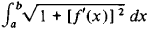The volume of the solid generated by rotating this arc about the x-axis is expressed by the integral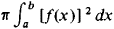and the surface area of that solid by the integral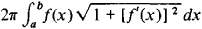The actual computation of definite integrals is accomplished by various methods. In certain cases the definite integral may be found by directly computing the limit of the corresponding integral sums. However, for the most part, such a passage to the limit is cumbersome. Some definite integrals may be computed by finding an indefinite integral first (see below: Indefinite integral). But as a rule, it is necessary to resort to an approximate calculation of definite integrals using various quadrature formulas (for example, the trapezoidal rule, Simpson’s rule). Such approximate calculations may be carried out on a computer with an absolute error not exceeding any given small positive number. In cases not requiring great accuracy, graphic methods are used for the approximate calculation of definite integrals.

The concept of the definite integral is extended to the case of unbounded intervals of integration as well as to certain classes of unbounded functions. Such generalizations are called improper integrals.

Expressions of the form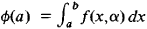where the function f(x,a) is continuous in x, are said to be parameter-dependent integrals. They serve as fundamental means for studying many special functions (for example, the gamma function).

Indefinite integral. The finding of indefinite integrals, or integration, is the inverse operation of differentiation. In differentiating a given function, we are seeking its derivative. In integration, we are seeking the antiderivative (or primitive) function—a function whose derivative is equal to the given function. Thus the function F(x) is an antiderivative for a given function f(x) if F’(x) = f(x) or, which is the same thing, if dF(x) = f(x) dx. A given function f(x) may have different an-tiderivatives, but all of these differ from one another only by a constant. Therefore, all antiderivatives of f(x) are contained in the expression F(x) + C, which is called the indefinite integral of the function f(x) and is written as

f(x) dx = F(x) + C

The definite integral viewed as a function of the upper limit of integration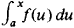(“integral with a variable upper limit”) is one of the antiderivatives of the integrand. This yields the fundamental formula of the integral calculus (the Newton-Leibniz formula):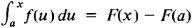which expresses the numerical value of the definite integral as the difference of the values of any antiderivative of the integrand at the upper and lower limits of integration.

The mutually inverse character of the operations of integration and differentiation is expressed by the equalities

d∫f(x) dx = f(x) dx ∫dF(x) = F(x) + C

This leads to the possibility of obtaining formulas and rules for integration from corresponding formulas and rules for differentiation (see Table 1, where C, m, a, and k are constants and m ≠ – 1 and a > 0).

Unlike derivatives, integrals of elementary functions cannot always be expressed in terms of elementary functions; they cannot be expressed “in closed form.” Each of the available methods of integration in closed form has a limited area of applicability (integration methods are set forth in textbooks on mathematical analysis; comprehensive tables of integrals are given in many handbooks).

The class of functions whose integrals can be expressed through elementary functions includes the rational functions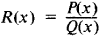where P(x) and Q(x) are polynomials. Many functions that are not rational are also integrable in closed form, for example, functions rationally dependent on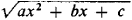and x or on x and rational powers of (ax + b)/(cx + d). Many transcendental functions are also integrable in closed form, for example, rational functions of sine and cosine. Functions represented by indefinite integrals that cannot be expressed in closed form are new transcendental functions. Many of these have been studied in detail (for example, the logarithmic integral, sine integral, cosine integral, exponential integral).

The concept of the integral can be extended to functions of several real variables as well as to functions of a complex variable and to vector functions.

(SeeINTEGRAL for extensions and generalizations of the concept of the integral.)

Historical notes. The integral calculus arose in connection with problems of determining areas and volumes. A number of problems of this kind were solved by ancient Greek mathematicians, who anticipated the ideas of the integral calculus to a significantly greater degree than those of the differential calculus. A large role in the solution of such problems was played by the method of exhaustion, which was established by Eudoxus of Cnidus and was used widely by Archimedes. However, Archimedes did not isolate the common content of the methods of integration or the concept of the integral and, furthermore, did not establish the algorithms of the integral calculus. The scholars of the Middle and Near East in the ninth to 15th centuries studied and translated the works of Archimedes into Arabic but did not obtain substantially new results in the area of the integral calculus. The work of European scholars during this time was even more modest. Only in the 16th and 17th centuries did the development of natural science in Europe give rise to new problems, in particular, problems on determining quadratures, cuba-tures, and centers of gravity. The works of Archimedes, first published in 1544 (in Latin and Greek), began attracting wide attention, and their study was one of the most important starting points for the further development of the integral calculus. The classical method of “indivisibles” was revived by J. Kepler. In more general form, the ideas behind this method were developed by B. Cavalieri, E. Torricelli, J. Wallis, and B. Pascal. A number of geometric and mechanical problems were solved by the method of indivisibles. To this same period belong the subsequently published works of P. Fermat on the quadrature of parabolas of the nth degree and later the works of C. Huygens on the rectification of curves.

As a result of these studies, the common features of the methods of integration used in the solution of outwardly dissimilar geometric and physical problems became apparent. This led to quadratures being viewed as the geometric equivalent of the definite integral. The final link in the chain of discoveries of this period was the establishment of the mutually inverse connection between problems of tangent construction and quadrature problems, that is, between differentiation and integration. The principal concepts and algorithms of the integral calculus were formulated independently by Newton and Leibniz. The term “integral calculus” and the designation of the integral by ∫ ydx were introduced by Leibniz.

Moreover, the concept of the indefinite integral (fluents) played a fundamental role in Newton’s works, whereas Leibniz proceeded from the concept of the definite integral. The further development of the integral calculus in the 18th century was the work of Johann Bernoulli and especially L. Euler. At the beginning of the 19th century, A. Cauchy based the integral and differential calculus on the theory of limits. The Russian mathematicians M. V. Ostrogradskii, V. Ia. Buniakovskii, and P. L. Chebyshev contributed to the development of the integral calculus in the 19th century. By the 20th century, the development of set theory and the theory of functions of a real variable led to a deepening and generalization of the fundamental concepts of the integral calculus as represented in the work of B. Riemann, H. Lebesgue, and others.

### REFERENCES

History
Van der Waerden, B. L. Probuzhdaiushchaiasia nauka. Moscow, 1959. (Translated from Dutch.).
Wileitner, H. Istoriia matematiki ot Dekarta do serediny 19 stoletiia, 2nd ed. Moscow, 1966. (Translated from German.)
Struik, D. J. Kratkii ocherk istorii matematiki, 2nd ed. Moscow, 1969. (Translated from German.)
Cantor, M. Vorlesungen über Geschichte der Mathematik, 2nd ed., vols. 3–4. Leipzig-Berlin, 1901–24.
Works by the founders and classical figures of integral calculus
Newton, I. Matematicheskie raboty. Moscow-Leningrad, 1937. (Translated from Latin.)
Leibniz, G. von. “Izbrannye otryvki iz matematicheskikh sochinenii.” Uspekhi matematicheskikh nauk, 1948, vol. 3, issue 1. (Translated from Latin.)
Euler, L. Integral’noe ischislenie, vols. 1–3. Moscow, 1956–58. (Translated from Latin.)
Cauchy, A. L. Kratkoe izlozhenie urokov o differentsial’nom i integral’ nom ischislenii. St. Petersburg, 1831. (Translated from French.)
Cauchy, A. L. Algebraicheskii analiz. Leipzig, 1864. (Translated from French.)
Textbooks and handbooks on integral calculus
Khinchin, A. Ia. Kratkii kurs matematicheskogo analiza, 3rd ed., 1957.
Smirnov, V. I. Kurs vysshei matematiki, 22nd ed., vol. 1. Moscow, 1967.
Fikhtengol’ts, G. M. Kurs differenlsial’nogo i integral’nogo ischisleniia, 7th ed., vol. 2. Moscow, 1969.
Il’in, V., and E. G. Pozniak. Osnovy matematicheskogo analiza, 3rd ed., part 1. Moscow, 1971.
Courant, R. Kurs differentsial’nogo i integral’nogo ischisleniia, 4th ed., vol. 1. Moscow, 1967. (Translated from German and English.)
Dwight, H. B. Tablitsy integralov i drugie matematicheskie formuly. Moscow, 1964. (Translated from English.)
A. N. KOLMOGOROV

## integral calculus

[′int·ə·grəl ′kal·kyəl·ləs]
(mathematics)
The study of integration and its applications to finding areas, volumes, or solutions of differential equations.
McGraw-Hill Dictionary of Scientific & Technical Terms, 6E, Copyright © 2003 by The McGraw-Hill Companies, Inc.

## integral calculus

the branch of calculus concerned with the determination of integrals and their application to the solution of differential equations, the determination of areas and volumes, etc.
Collins Discovery Encyclopedia, 1st edition © HarperCollins Publishers 2005
References in periodicals archive ?
The same data were used to determine which formative assessment has the best impact on the students' summative assessment which is the departmental examination in Integral Calculus. These data collected were analyzed using mean, standard deviation, and Pearson product measurement correlation and regression analysis.
This research aimed to: 1) determine the level of students' mathematics comprehension and previous mathematics performance; 2) determine the level of students' conceptual understanding of finding the area of plane regions in Integral Calculus; and 3) determine the impact of students' mathematics comprehension and previous mathematics performance on their conceptual understanding of finding the area of plane regions in Integral Calculus.
The text is highly technical, with many color maps, graphs, and illustrations, and equations utilizing up to differential and integral calculus.
of Barcelona) sets out the basic facts of linear functional analysis and its applications to some fundamental aspects of mathematical analysis, for graduate students of mathematics familiar with general topology, integral calculus with Lebesgue measure, and elementary aspects of normed or Hilbert spaces.
One central idea of integral calculus is that both the Riemann Lower Sum, and the Riemann Upper Sum are approximations for the area under the graph of y = f( x) (see  and ):
There are many applications of integral calculus and developing a deeper understanding of some of the numerical methods will increase understanding of the techniques.
There are four chapters: The Nature of the Infinite Process, The Definite Integral, Differential and Integral Calculus, and Applications to Problems of Motion.
PC Macsyma performs basic and matrix algebra and trigonometry, differential and integral calculus, and vector and tensor analysis.
Authors Morris and Stark present students and academics with a comprehensive introduction to both differential and integral calculus using real-world examples from a variety of fields, including entrepreneurship, economics, environmental science, and the social sciences.
Kuhfittig (Milwaukee School of Engineering) offers a textbook introducing differential and integral calculus within a two-year technology program, but says it could also be used along with others in a four-year program.
(For some discussion of the relative importance of the algorithmic approach to learning and the conceptual approach, see Pettersson and Scheja (2008).) Secondly, the formal manipulations required for high school differential calculus questions are simpler than those for integral calculus.
This intermediate-level textbook explains probability and statistics to undergraduate and graduate students in engineering, physical sciences, and management who have knowledge of college algebra, differential and integral calculus, and some linear algebra concepts.

Site: Follow: Share:
Open / Close